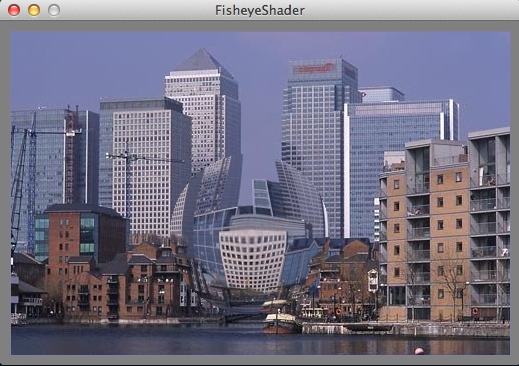#### Howdy, Stranger!

We are about to switch to a new forum software. Until then we have removed the registration on this forum.

#### In this Discussion

edited December 2016Sample output from the sketch below

For a long time I have been meaning to investigate shaders with the idea of creating my own. Yesterday I took the plunge and started messing about with the examples that came with Processing. I decided that a good start would be a fisheye lens shader and here is my effort for you to play with.

Sketch code

``````PImage img;
int offsetX, offsetY;

public void setup() {
size(520, 344, P2D);
noCursor();
// The offsets are needed if the image size is
// different from the display size
offsetX = (width - img.width)/2;
offsetY = (height - img.height)/2;
}

public void mouseMoved() {
shade.set("lensPos", mouseX - offsetX, mouseY - offsetY);
}

public void draw() {
background(128);
drawOutput(offsetX, offsetY, img.width, img.height);
}

}

public void drawOutput(float x, float y, float w, float h) {
pushMatrix();
translate(x, y);
vertex(0, 0, 0, 0);
vertex(0, h, 0, 1);
vertex(w, h, 1, 1);
vertex(w, 0, 1, 0);
endShape();
popMatrix();
}
``````

Use a text editor to create this file and put it in the sketch folder.

``````uniform sampler2D image;

// Image size in pixels
uniform ivec2 size;

// Lens position
uniform ivec2 lensPos;

// Lens size (radius in pixels)
uniform float lensSize;

varying vec4 vertTexCoord;

void main() {
// Get current uv position
vec2 st = vertTexCoord.st;

// get current pixel position
vec2 pxl = size * st;

// Get distance from lens centre
float pxl2lens = distance(pxl, lensPos);

// If outside lens use pixel
if(pxl2lens > lensSize){
gl_FragColor = texture2D(image, st).rgba;
return;
}
// We are inside the lens radius so calculate
// new uv coordinate

// Get direction vector to pixel
vec2 direction = pxl - lensPos;

float factor = 0.4 + 0.9 * pxl2lens / lensSize;
vec2 npxl = lensPos + direction * factor;
vec2 nst = npxl / size;

gl_FragColor = texture2D(image, nst).rgba;
}
``````
Tagged: